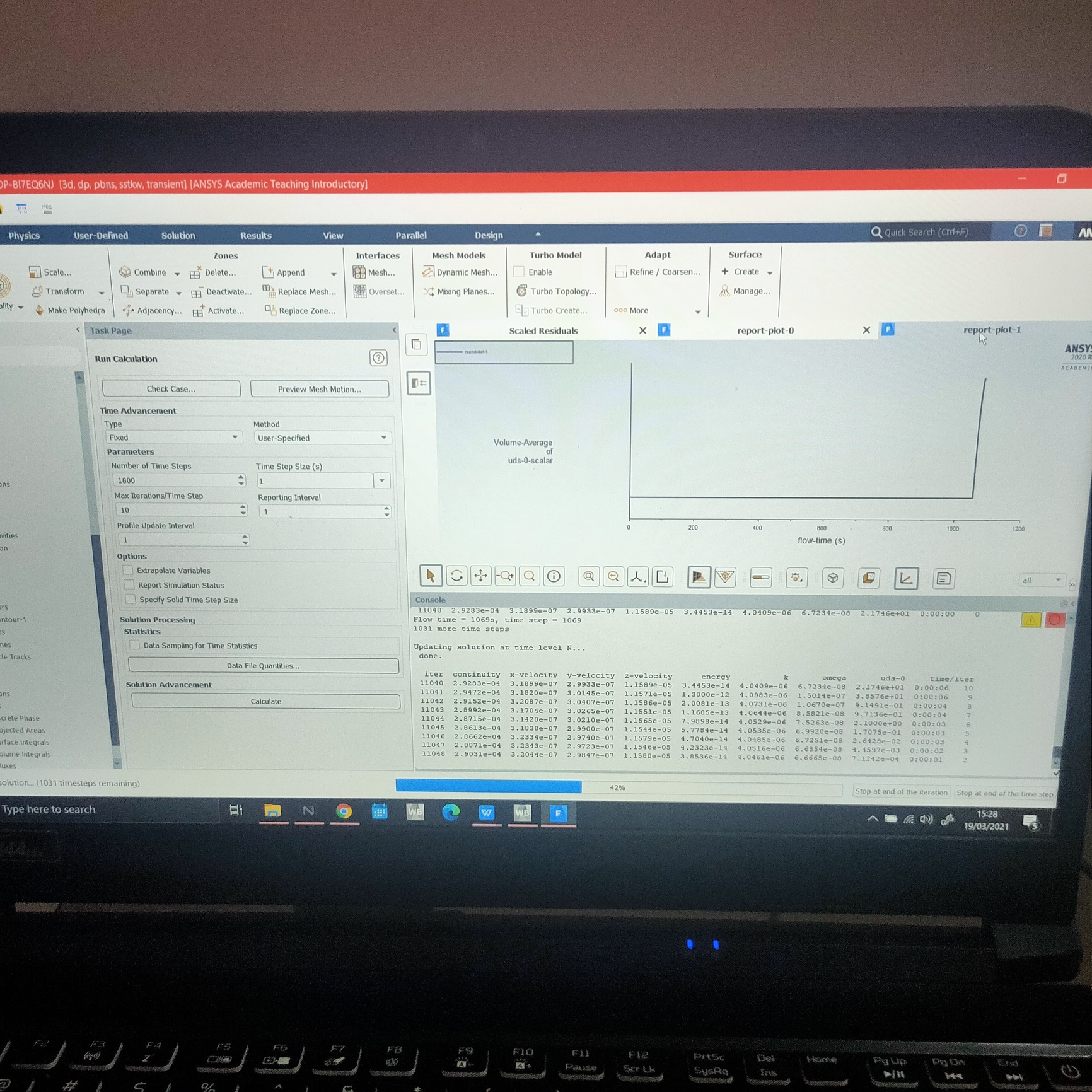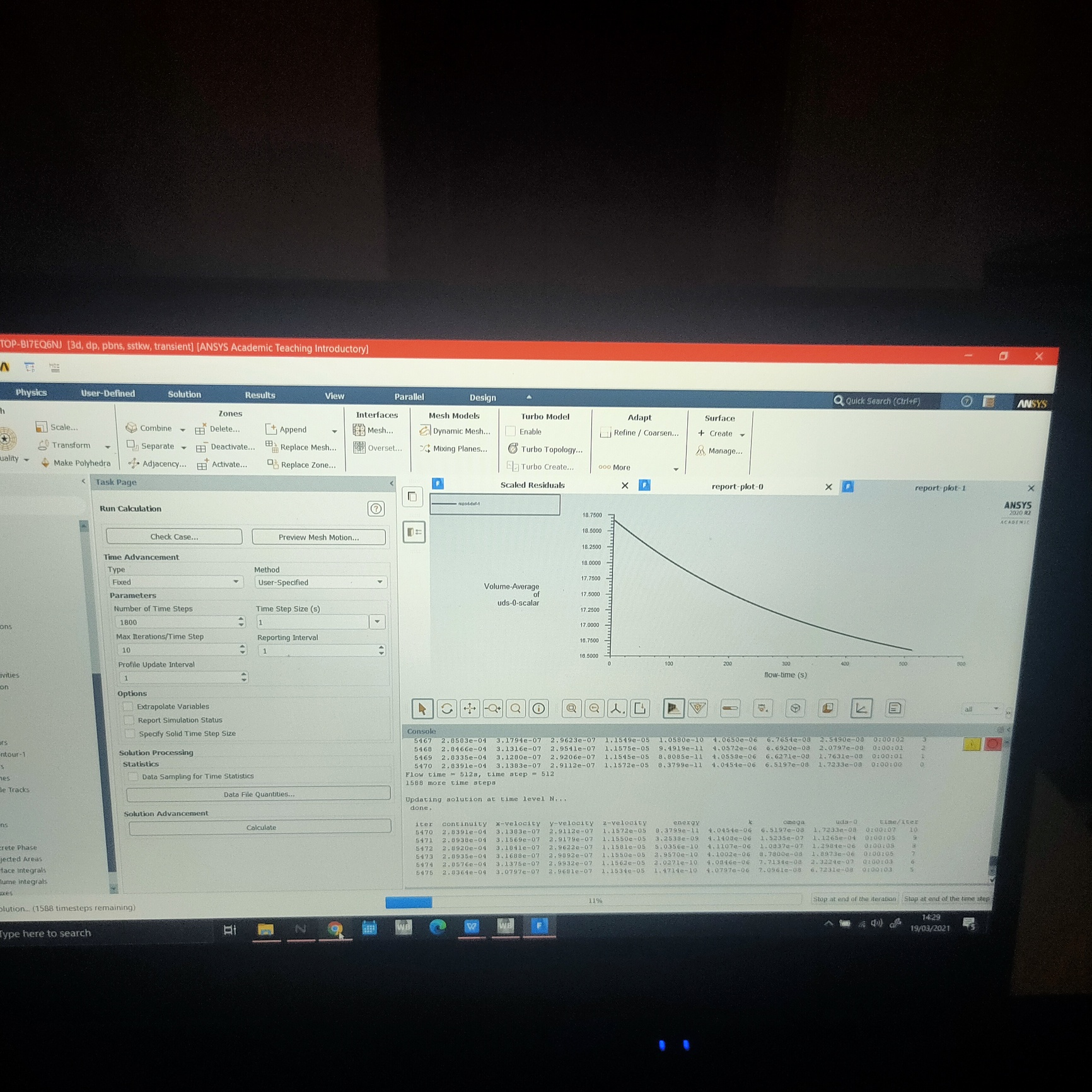Alvin
SubscriberThank you for replying nI'm using Ansys Student 2020 R2, I used expressions to create the mass transfer equation instead of using UDF. I used a converged steady state solution before modelling transient heat and mass transfer. The simulation solves for continuity, energy, turbulence (bcoz of SST k-omega model) and UDS. I monitored the simulation by plotting the vol average of Scalar 0 (moisture content) over time. As you can see in the pictures, it started fine, showing exponential trend, then after some time, the trend changes into an absurd one. What could be the reason behind this??n